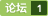求教MATLAB如何做递归？ [问题点数：40分]/*1.一个人赶着鸭子去每个村庄卖，每经过一个村子卖去所赶鸭子的一半又一只。 这样他经过了七个村子后还剩两只鸭子，问他出发时共赶多少只鸭子？经过 每个村子卖出多少只鸭子?*/#include int q(int duck,int village)//定义<em>递归</em>函数，参数为鸭子数和村子数 { if(village==0) //未经过村子时的鸭子为总数 printf("总共有%d个鸭子。\n
matlab递归生成树

C#写COM组件
<em>求教</em><em>求教</em><em>求教</em><em>求教</em><em>求教</em><em>求教</em><em>求教</em>

function [ output_args ] = perm( list , s  ,e  ) %UNTITLED4 Summary of this function goes here %   Detailed explanation goes here if( s ==  e )      disp( num2str( list ) ); else     for( i = s

MATLAB递归函数
MATLAB<em>递归</em>函数 <em>递归</em>函数就是函数调用自己本身，具体来说就是在一个函数模块里（设函数模块为factor.m，），存在函数（factor），这个函数（factor）用来调用函数模块（factor.m）。看下面的例子： <em>递归</em>函数求n的阶层 创建函数文件factor.m function f=factor(n) % function 输出形参表 = 函数名（输入形参表）； if n&lt...

matlab递归迭代思路

matlab递归实现汉诺塔m函数文件(动画演示)

RQAmatlab工具箱

【算法】快速傅里叶变换（FFT）的递归实现
FFT是数字信号处理中的重要算法，在<em>matlab</em>中可以直接调用fft()函数，本文是C++版的FFT算法，用<em>递归</em>方式进行实现。 //================================== //Signal_Process_FFT_v1.cpp //================================== #include&amp;lt;iostream&amp;gt; #inclu...

<em>递归</em>算法： double determinant(double **matrix,int dim) { double result = 0; int i,k; double **subMatrix = (double **) new double*[dim]; for(i = 0; i < dim; i++) subMatrix[i] = (double *) new double[

<em>递归</em>神经网络的条件随机场 Conditional random fields as recurrent neural networks (2015) 作者S. Zheng and S. Jayasumana. 记忆网络 Memory networks (2014) 作者J. Weston et al. 神经网络图灵机 Neural turing machines (2014) 作者A. Graves et al. <em>递归</em>神经网络生成序列 Generating sequences with recurrent neural networks (2013) 作者A. Graves.
matlab 使用递归方法列出或查找指定目录下的文件
%调用格式： % %[pathstruct,pathstr]=listfiles('.\','.csv') % %[pathstruct,pathstr]=listfiles('d:\myfiles','.bmp')

RLS算法中，权矢量随迭代次数的增长而变化的收敛情况

copy文件中指定 文本类型文件（*.txt, *.c, *.h等文件），支持子目录递归拷贝，用 matlab 实现。
srcDirName = 'F:\my_source_direction\';destDirName = 'F:\my_destination_direction\';% suffix = 'txt'; % suffix = 'cpp'; % suffix = 'c'; suffix = 'h'; % suffix = 'hcpp'; myCopyFileAndSubDir(srcDirName,

005E3887 >  68 EC86995F     push 0x5F9986EC 005E388C    E8 57090800     call 复件_悍?006641E8 005E3891    68 EC943E60     push 0x603E94EC 005E3896    E8 8B020800     call 复件?00663B26 005E389B    6D

P1028 数的计算（洛谷递归

《Recursive implementation of the Gaussian filter》一文实现了一种（infinite impulse response）IIR型的高斯滤波器，基于<em>递归</em>结构，在每维度只有6次乘加运算，与高斯核大小无关。

function y = fib(  n ) %UNTITLED2 Summary of this function goes here %   Detailed explanation goes here if ( n == 1 || n==  2 )     y=1;     return   ;   else   y = fib(n -1)  +  fib( n -2 ) ;

<em>求教</em>一道关于求解最优化问题的编程问题~

fram实例fram实例fram实例fram实例fram实例

<em>如何做</em>前端监控系统<em>如何做</em>前端监控系统<em>如何做</em>前端监控系统<em>如何做</em>前端监控系统<em>如何做</em>前端监控系统<em>如何做</em>前端监控系统

-

vb-mschart查询Access数据
vb-mschart查询Access数据， 顺便<em>求教</em>。问题在里面。 noleic@foxmail.com 谢谢

FFT快速傅里叶变换-递归版-带注释模板
FFT 真·存模板系列

<em>递归</em>生成全排列算法： void perm2(int *arg,int n,int k) { int i; if(k&amp;gt;=n) { //print } else { for(i = k;i &amp;lt; n;++i) { swap(arg[i],arg[k]); perm1(arg,n,k+1); swap(arg[i],arg[k]); ...

Sierpinski“垫片”的构造原理如下：首先取一个正三角形，将其等分为四个小的正三角形，并舍去中间的一个，然后将余下3个正三角形中的每一个再等分为4个更小的正三角形，并舍去各自中间的一个，进一步再将余下的9个正三角形分别按同样的方法操作取舍，如此反复操作下去，直至无穷。最后所得图形就是谢尔宾斯基三角形，亦即Sierpinski“垫片”。由上述原理可知，该图形具有严格的相似性，可通过递

GL音乐播放器--代码实现--实现换肤功能

LSTM和递归网络基础教程

<em>递归</em>求解几类排列组合问题，求组合数列的情况

FMG运行图 MATLAB<em>递归</em>代码 clear; clc; global f1 f1 = fopen('out1.txt', 'w'); %该文件只是为了测试 k = 5; ks = 1; % 总的网格层、开始层 gamma = 1; ncy = 1; % 类型(V or W), 总的循环次数 nu0 = 6; nu1 =2; nu2 = 1; fmg(k, ks, nu0, nu1, n...
Matlab 教程

<em>如何做</em>优盘的启动盘<em>如何做</em>优盘的启动盘<em>如何做</em>优盘的启动盘

/** * 求阶乘：利用<em>递归</em>调用实现 * @author * */ public class FactorialDemo { public static void main(String[] args) { System.out.println(“5的阶乘为:”+getResult(5)); } public static int...

PS 教程 图层
ＰＳ教程，赶紧的，需要的，<em>求教</em>的　　拿走吧

<em>递归</em>就是直接或间接调用自身。算法思想：原问题可分解子问题（必要条件），原与分解后的子问题相似（<em>递归</em>方程），分解次数有限（子问题有穷），最终问题可直接解决（<em>递归</em>边界），经典问题有：幂运算、阶乘、组合数、斐波那契数列、汉诺塔等。这里详细介绍： 快速幂的实现； 斐波那契数列的矩阵加速实现；
【神经网络篇】--RNN递归神经网络初始与详解

package gxx20;import java.util.Scanner;//斐波那契数列：第n个月有m只兔子//1,1,2,3,5,8,13,21...public class FeiBoNaQieDemo { public static void main(String[] args) { Scanner scanner = new Scanner(System.in); System...
matlab图像的邻域操作与块操作
1.图像的滑动邻域操作。    邻域操作是指将每个输入的像素值以及其某个邻域的像素值结合处理而得到对应的输出像素值的过程。邻域通常形状规则。如2*2，2*3之类。滑动邻域操作一次处理一个像素。   对于m*n的邻域，中心像素坐标为：floor（([m,n]+1)/2）；  例如：邻域2*2——>[1 1]        邻域2*3——>[1 2]  实现滑动邻域操作的步骤：    (1) 选择一个单独的像素。<b
MATLAB遍历子文件夹及其下文件
MATLAB遍历子文件夹及其下文件以前从未遇到过用MATLAB批处理文件的情况，此次项目需要批量将二进制数据文件导入<em>matlab</em>进行svm分类，将<em>matlab</em>遍历子文件夹及其文件的方法记录下来。文件目录结构/maindir |-- subdir1 |-- datafile1.dat |-- datafile2.dat |-- subdir2

#include #include #define LENGTH 9 int answer = 0; void printSudo(int array[][LENGTH]) {     printf("\n\n");     int i, j;     for (i = 0; i < LENGTH; i++) {         if ((i + 1) % 3 == 0)

《超越对手--软件项目经理的18种实用技能》
1.前言 2.<em>如何做</em>业务调研 3.如何写解决方案 4.<em>如何做</em>产品演示 5.<em>如何做</em>用户考察 6.<em>如何做</em>公司介绍 7.培训工作 8.<em>如何做</em>现场推广 9.<em>如何做</em>项目验收 10.<em>如何做</em>项目团队管理

<em>求教</em>类似SUMIF;COUNTIF的条件函数的写法？？？

fano编码的递归算法
fano编码的<em>递归</em>算法
Matlab 递归求解八皇后问题

import java.awt.Color; import java.awt.FlowLayout; import java.awt.Font; import java.awt.Graphics; import java.awt.event.ActionEvent; import java.awt.event.ActionListener; import javax.swing.JButton;
Hilbert空间递归演示
Hilbert空间填充曲线在图像采样等方面十分有用关于什么希尔伯特 空间填充曲线看这里：http://en.wikipedia.org/wiki/Hilbert_curve

#include #include #include #include const int mx_n = 4e5 + 10; const double pi = acos(-1.0); #define rep(i, s, t) for(register int i = s; i <= t; ++i)using namespac

#1~10的累加和==10+(1~9的累加和) # result = 1+2+3+4+5+6+7+8+9+10 def s(n): if n==1: return 1 result = n+s(n-1) return result if __name__ == '__main__': result = s(10) #...